# Zero counting functions

Below we present the standard notation for the functions which count zeros of the zeta-function.

Zeros of the zeta-function in the critical strip are denoted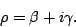It is common to list the zeros with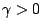in order of increasing imaginary part as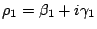,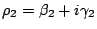,.... Here zeros are repeated according to their multiplicity.

We have the zero counting function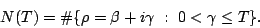In other words,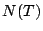counts the number of zeros in the critical strip, up to height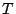. By the functional equation and the argument principle,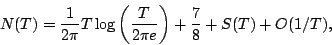where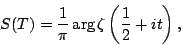with the argument obtained by continuous variation along the straight lines from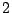to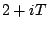to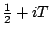. Von Mangoldt proved that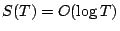, so we have a fairly precise estimate of the number of zeros of the zeta-function with height less than. Note that Von Mangoldt's estimate implies that a zero at heighthas multiplicity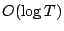. That is still the best known result on the multiplicity of zeros. It is widely believed that all of the zeros are simple.

A number of related zero counting functions have been introduced. The two most common ones are: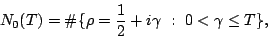which counts zeros on the critical line up to height. The Riemann Hypothesis is equivalent to the assertion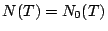for all. Selberg proved that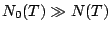. At present the best result of this kind is due to Conrey [ MR 90g:11120], who proved that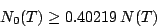ifis sufficiently large.

And,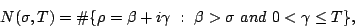which counts the number of zeros in the critical strip up to height, to the right of the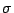-line. Riemann Hypothesis is equivalent to the assertion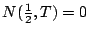for all.

For more information on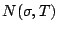, see the article on the density hypothesis.

Back to the main index for The Riemann Hypothesis.